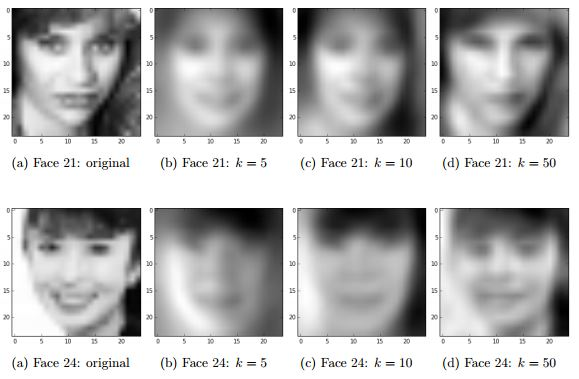# Eigen-faces

Languages: For this project I used Python 2.7 and the scipy stack. I specifically used numpy to handle matrices and the calculations on those matrices and matplotlib to plot the reconstructed faces.

Overview: In this project, I was given an image of a grayscale face. My task was to first reduce the dimensions of the face using singular value decomposition (SVD). I then used principal components analysis (PCA) to reconstruct a face in this lowered dimension using the first K principal directions.

Results: When taking the first K = [5,10,50] principal directions on two randomly chosen faces, we can achieve a pretty good reconstruction result when k = 50. Below are the results when using the first K principal directions.